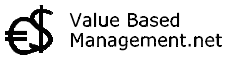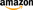## IRR CalculationThe Internal Rate of Return (IRR) is the discount rate that results in a net present value of zero for a series of future cash flows. It is an Discounted Cash Flow (DCF) approach to valuation and investing just as Net Present Value (NPV). Both IRR and NPV are widely used to decide which investments to undertake and which investments not to make.   Calculation of IRR: The major difference is that while Net Present Value is expressed in monetary units (Euro's or Dollars for example), the IRR is the true interest yield expected from an investment expressed as a percentage.  Internal Rate of Return is the flip side of Net Present Value and is based on the same principles and the same math. NPV shows the value of a stream of future cash flows discounted back to the present by some percentage that represents the minimum desired rate of return, often your company's cost of capital. IRR, on the other hand, computes a break-even rate of return. It shows the discount rate below which an investment results in a positive NPV (and should be made) and above which an investment results in a negative NPV (and should be avoided). It's the break-even discount rate, the rate at which the value of cash outflows equals the value of cash inflows.   Many people find the percentages of IRR easier to understand than Net Present Value. Another benefit from IRR is that it can be calculated without having to estimate the (absolute) cost of capital. When IRR is used, the usual approach is to select the projects whose IRR exceeds the cost of capital (often called hurdle rate when used in the IRR context). This may seem simple and straightforward at first sight. However a major disadvantage of using the Internal Rate of Return instead of Net Present Value is that if managers focus on maximizing IRR and not NPV, there is a significant risk in companies where the return on investment is greater than the Weighted Average Cost of Capital (WACC) that managers will not invest in projects expected to earn greater than the WACC, but less than the return on existing assets. IRR is a true indication of a project's annual return of investment only when the project generates no interim cash flows - or when those interim investments can be invested at the actual IRR. The aim of the value-oriented manager should be to invest in any project that has a positive NPV! If IRR usage is unavoidable, then managers are advised to use so called Modified IRR (which, while not perfect, at least allows to set more realistic interim reinvestment rates) and additionally to keep a close look on interim cash-flows, especially if they are biased to the beginning of the project period (the distortion is bigger then). In other words: the aim should not be to maximize the Internal Rate of Return, but to maximize Net Present Value. Book: Aswath Damodaran - Investment Valuation: Tools and Techniques for Determining the Value of Any Asset -Book: James R. Hitchner - Financial Valuation: Applications and Models -Book: Steven M. Bragg - Business Ratios and Formulas : A Comprehensive Guide -